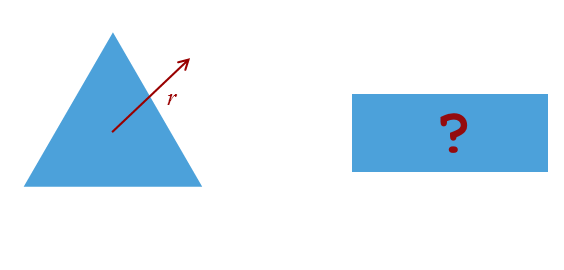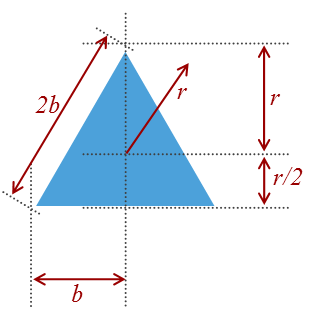﻿ Geometry Puzzle# Geometry Puzzle

Recently someone gave me a little geometry puzzle:

There is an equilateral triangle inscribed in a circle (image on left). What would be the dimensions (height, width) of an inscribed rectangle that has the same area as the triangle?(When the puzzle was described to me, it was quoted that the circle had a radius of two units, but I’m going to simply use r here to get a generic solution).

At first glance, it looked like it would be a fairly trivial puzzle to solve, and one that, when you saw the answer, it would just look ‘right’ and obvious. As I got into solving it, however, it turned out to be a little trickier than I expected and the result did not jump out to me as being obvious.

If you want to play along, stop reading now and scribble a few attempts at solving.

### My solution

Below is the way I solved it. Somehow it doesn’t feel like a clean solution, and it seems over complicated. I know I’m tempting fate by publishing my solution here because I’m sure that, as soon as I do, someone is going to email me with a trivial way of solving it! Joking aside, if you can see an alternative (simpler) way of getting to the answer, please do email me.First of all some basics. The area of both shapes is A. We’ll define the height and width of the rectangle as x and y, so we can describe the area this way:Using Pythagoras, we can also generated an equation involving the radius. Because the rectangle is inscribed, the corners are touching the circle, and with symmetry, the hypotenuse is the diameter (twice the radius) of the circle:Next let’s look at the triangle. High school taught us that the area of a triangle is half the base multiplied by the vertical height. We’ll define the half the base as is b. You might have remembered from geometry class that the center of an inscribed equilateral triangle is one third of the height of the triangle, but if not, it’s easy to prove. The height of the triangle is the radius, plus the lower section. A triangle in this lower section has a hypotenuse of r and an angle from the vertical of 60°. cos(60°) = 0.5, and so the lower section has a vertical height of r/2. The total vertical height of the triangle is therefore 3r/2Next we need to calculate b. Using Pythagoras again:Combining (3) and (4) we get the area of the triangle in terms of the radius:We now have two equations (1) and (5), for the area of the polygons. We can square and equate these:Substituting in y2 from (2)Gives us this quartic:We can re-arrange this into a standard form quadratic of terms of x2Using the old-faithful quadratic equation formula:We get:A little simplification later we get to:But we want to find x, so we take the square root, and this gives us the two answers we need (height and width):### Results

The width of the rectangle is ≈ 1.876r and the height ≈ 0.692r (or vice versa).

I don’t know about you, but those results don’t look ‘obvious’ to me when we’re dealing with such simple geometric shapes!

You can find other articles on general geekery here.You can find a complete list of all the articles here.Click here to receive email alerts on new articles.